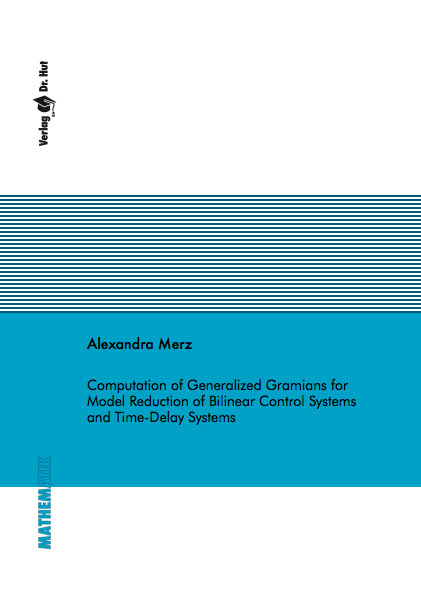Datenbestand vom 17. August 2019aktualisiert am 17. August 2019

# ISBN 9783843908443Euro 72,00 inkl. 7% MwSt

978-3-8439-0844-3, Reihe Mathematik

Alexandra Merz
Computation of Generalized Gramians for Model Reduction of Bilinear Control Systems and Time-Delay Systems

153 Seiten, Dissertation Technische Universität Kaiserslautern (2012), Softcover, A5

## Zusammenfassung / Abstract

In this thesis, efficient solution methods for generalized Lyapunov equations and delay Lyapunov equations are considered. Both problems will be dealt with in the context of model order reduction for bilinear control systems as well as for time-delay systems. For this classes of systems, neither a comprehensive theory nor numerical algorithms for computing efficiently the generalized Gramians, which are the solutions of the associated Lyapunov equations, exist.

Taking additional information from the underlying Carleman bilinearized systems into account, we can exploit the special matrix form to develop an iterative algorithm. This solution method is based on a Schur decomposition of the system matrices. Therefore, an efficient solution method for Kronecker matrix products is necessary and is derived.

Delay Lyapunov equations are matrix delay differential equations with symmetry and boundary conditions. Thus, the first developed iterative solution method solves a coupled system of ordinary differential equations. The second iterative solution method combines the solution of a Lyapunov equation with the solution of an ordinary differential equation. With the resulting delay Lyapunov matrix, it is possible to transfer the process of balanced truncation also to time-delay systems.